# RD Sharma Solutions for Class 6 Maths Chapter 14: Circles

We come across various objects in our daily lives which remind us of a Circle. Some of the examples of a circle are wheel, coin, full moon, bangles, tyres etc. We know that a circle has all the points in a plane with a fixed distance from a fixed point. The major concepts which are explained in this Chapter are angles, chords, arcs, segments and sectors.

Students who aspire to score good marks in the exam can download the solutions which are designed by the experts at BYJU’S. The primary aim of preparing solutions is to make learning fun for the students. RD Sharma Solutions for Class 6 Maths Chapter 14 Circles can be downloaded from the links which are provided here.

## RD Sharma Solutions for Class 6 Maths Chapter 14: Circles Download PDF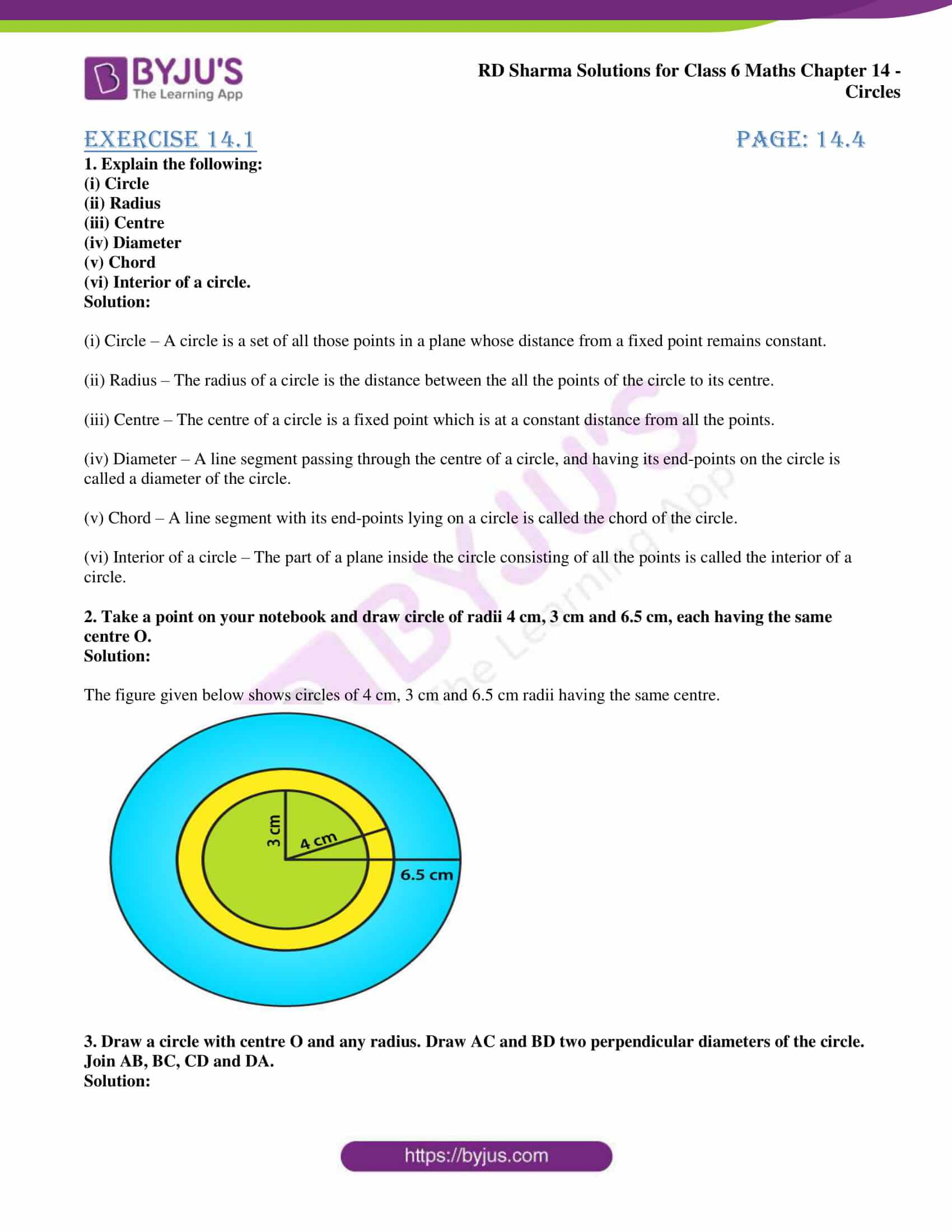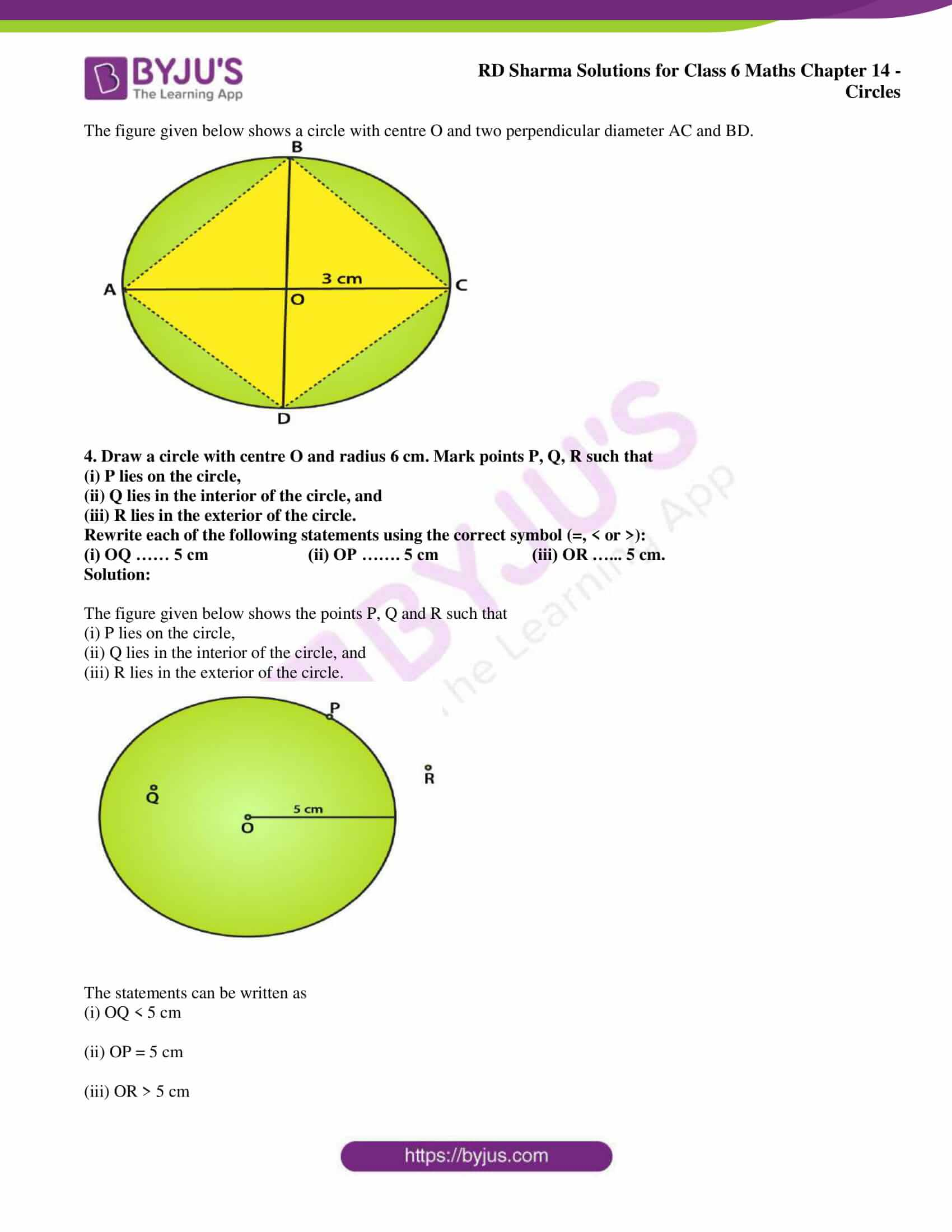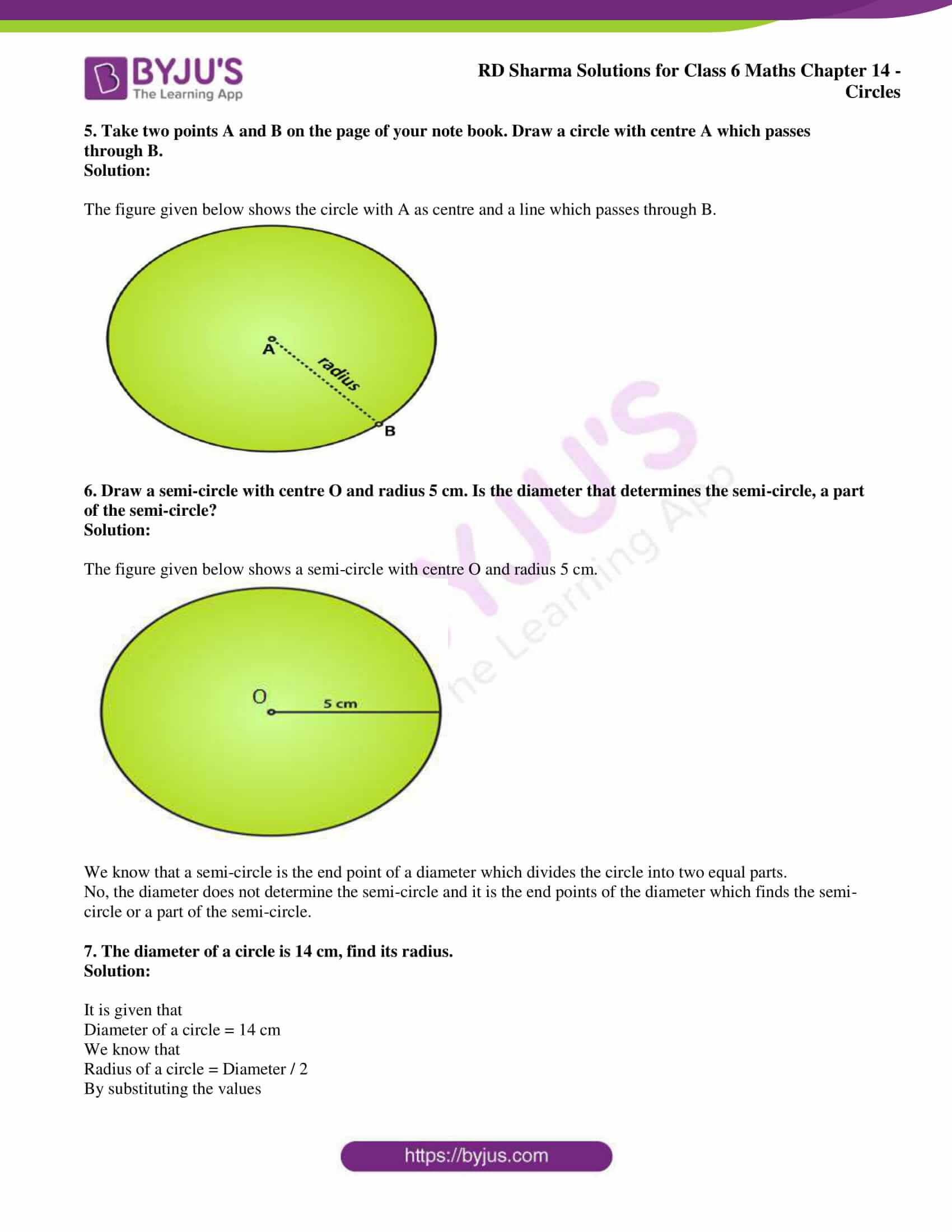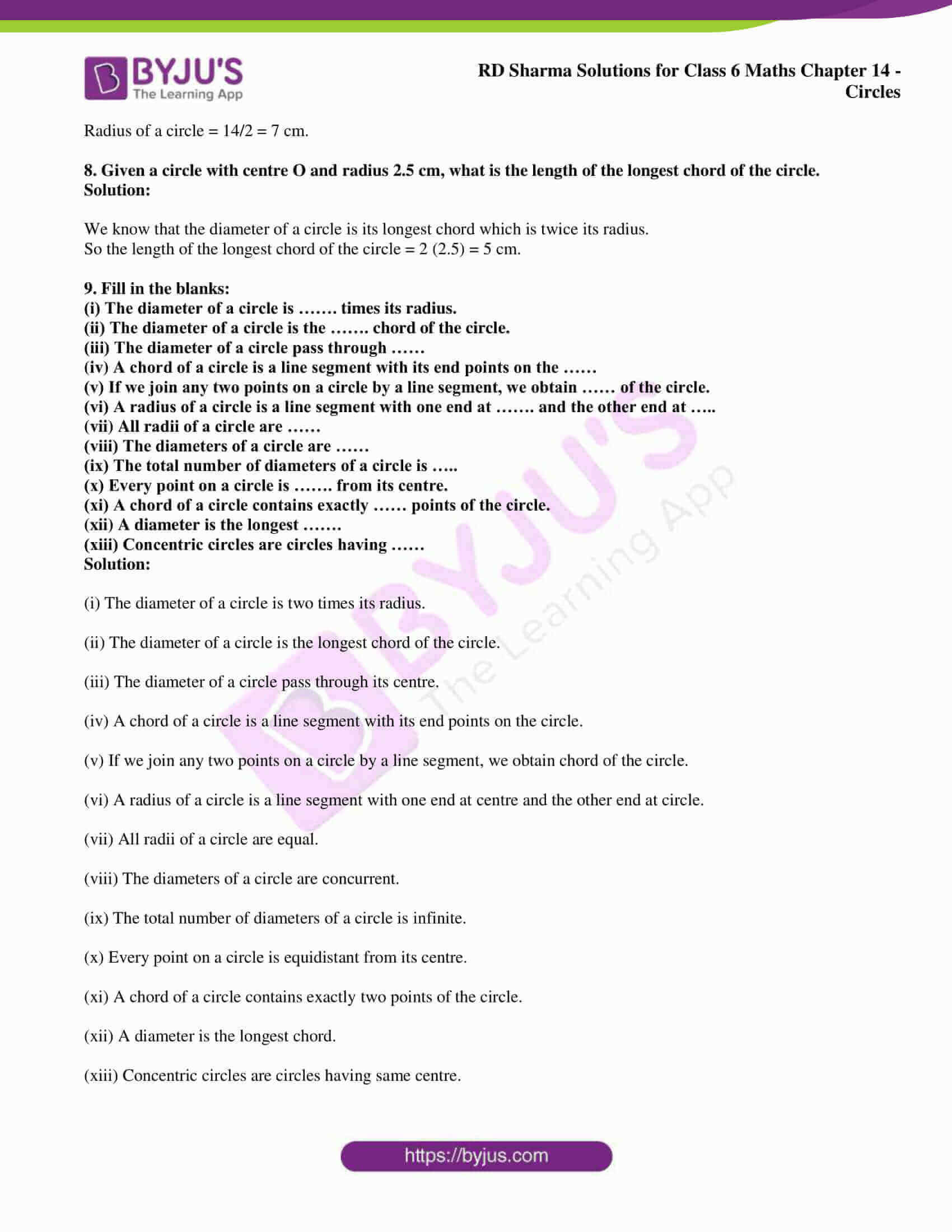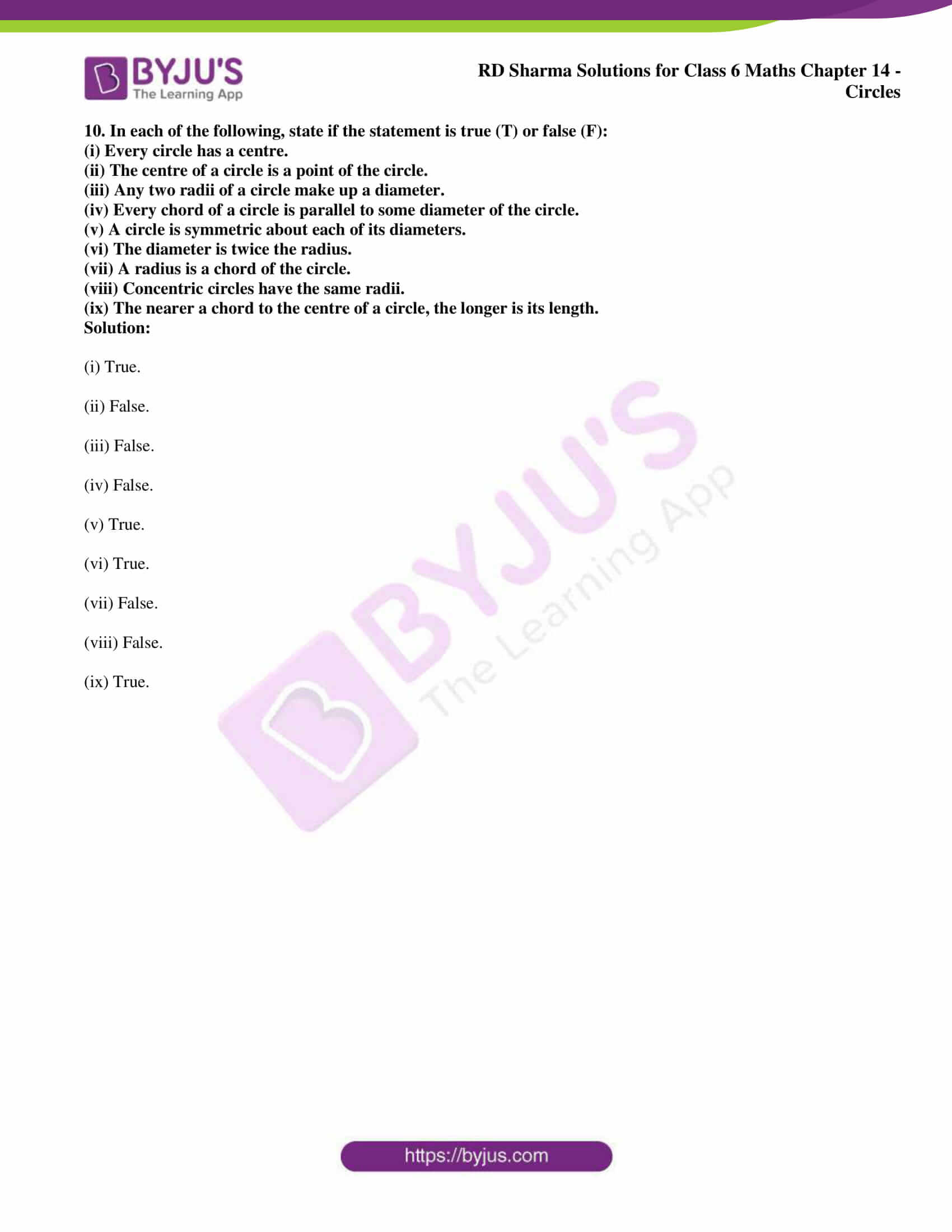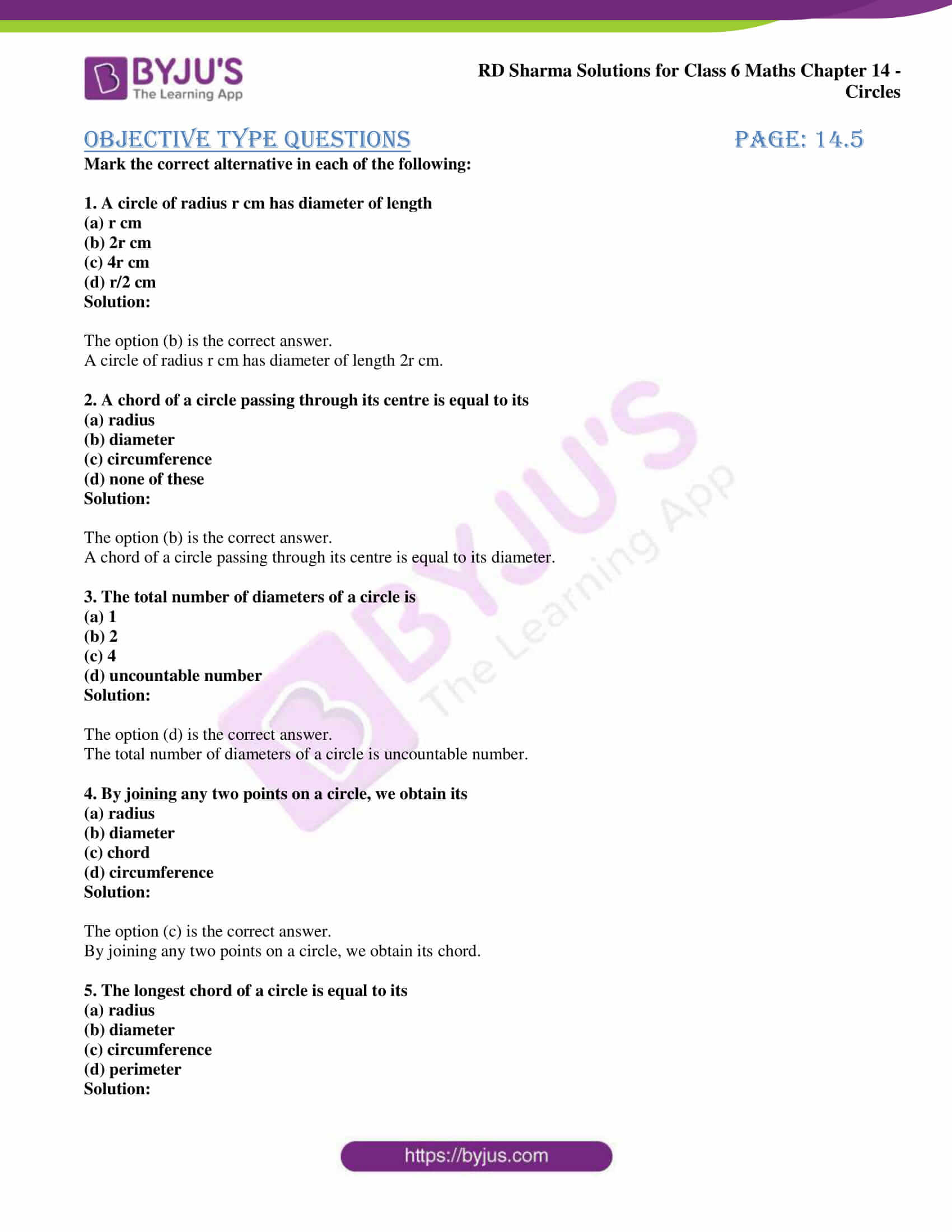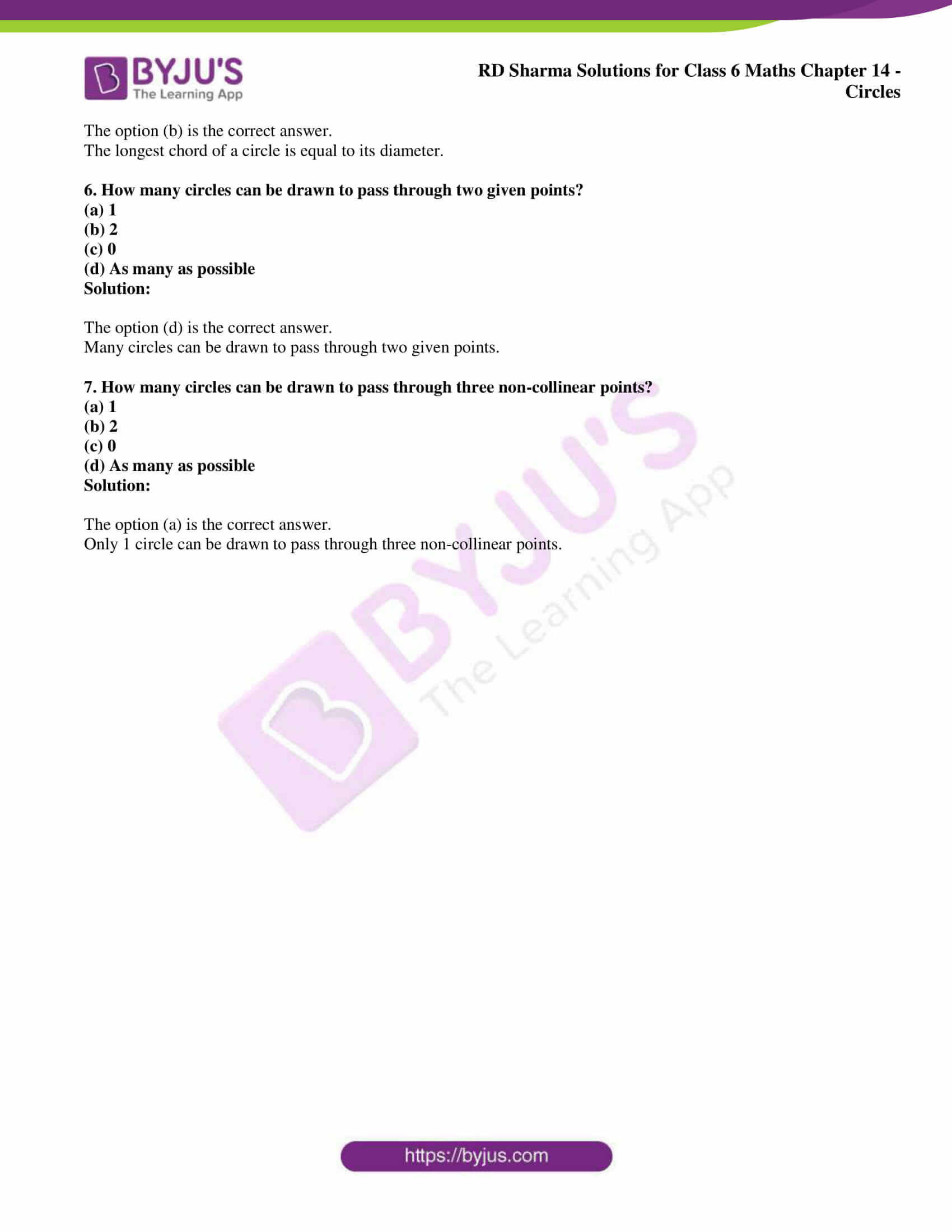## Access answers to Maths RD Sharma Solutions for Class 6 Chapter 14: Circles

### Exercise 14.1 page: 14.4

1. Explain the following:

(i) Circle

(iii) Centre

(iv) Diameter

(v) Chord

(vi) Interior of a circle.

Solution:

(i) Circle – A circle is a set of all those points in a plane whose distance from a fixed point remains constant.

(ii) Radius – The radius of a circle is the distance between the all the points of the circle to its centre.

(iii) Centre – The centre of a circle is a fixed point which is at a constant distance from all the points.

(iv) Diameter – A line segment passing through the centre of a circle, and having its end-points on the circle is called a diameter of the circle.

(v) Chord – A line segment with its end-points lying on a circle is called the chord of the circle.

(vi) Interior of a circle – The part of a plane inside the circle consisting of all the points is called the interior of a circle.

2. Take a point on your notebook and draw circle of radii 4 cm, 3 cm and 6.5 cm, each having the same centre O.

Solution:

The figure given below shows circles of 4 cm, 3 cm and 6.5 cm radii having the same centre.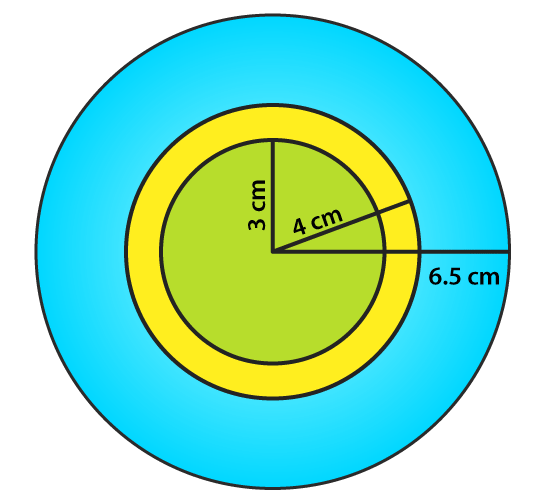3. Draw a circle with centre O and any radius. Draw AC and BD two perpendicular diameters of the circle. Join AB, BC, CD and DA.

Solution:

The figure given below shows a circle with centre O and two perpendicular diameter AC and BD.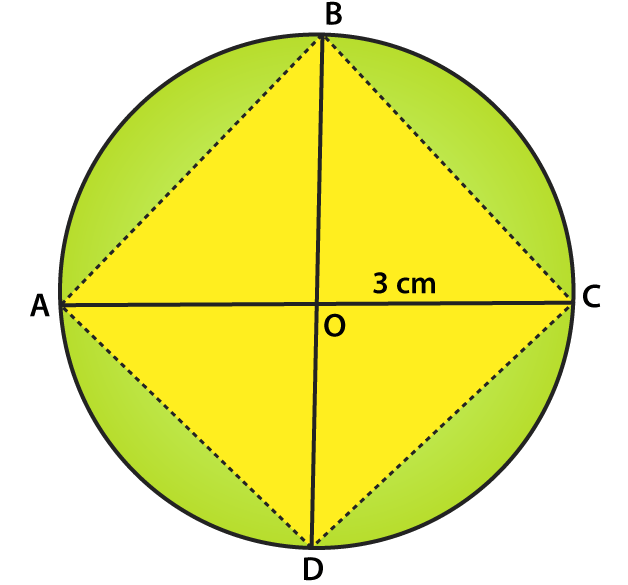4. Draw a circle with centre O and radius 6 cm. Mark points P, Q, R such that

(i) P lies on the circle,

(ii) Q lies in the interior of the circle, and

(iii) R lies in the exterior of the circle.

Rewrite each of the following statements using the correct symbol (=, < or >):

(i) OQ …… 5 cm (ii) OP ……. 5 cm (iii) OR …… 5 cm.

Solution:

The figure given below shows the points P, Q and R such that

(i) P lies on the circle,

(ii) Q lies in the interior of the circle, and

(iii) R lies in the exterior of the circle.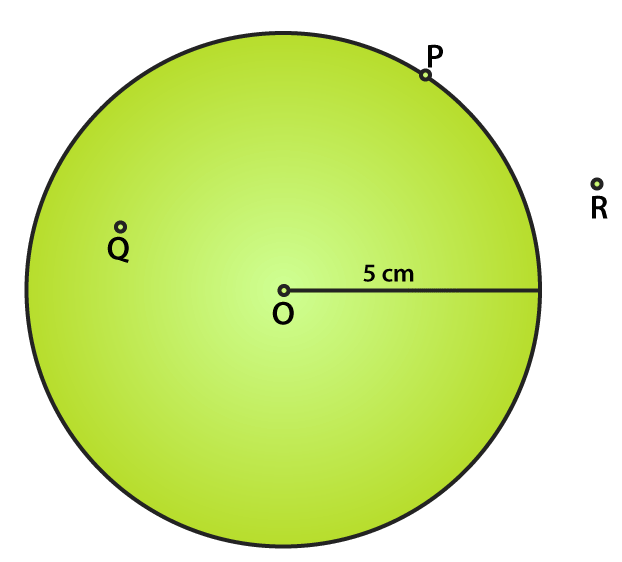The statements can be written as

(i) OQ < 5 cm

(ii) OP = 5 cm

(iii) OR > 5 cm

5. Take two points A and B on the page of your note book. Draw a circle with centre A which passes through B.

Solution:

The figure given below shows the circle with A as centre and a line which passes through B.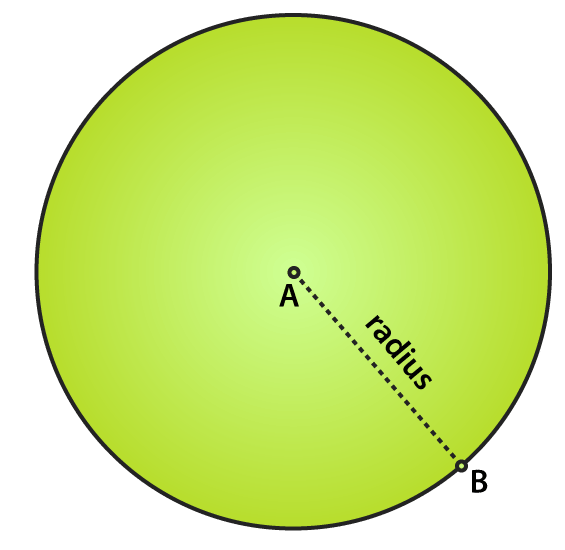6. Draw a semi-circle with centre O and radius 5 cm. Is the diameter that determines the semi-circle, a part of the semi-circle?

Solution:

The figure given below shows a semi-circle with centre O and radius 5 cm.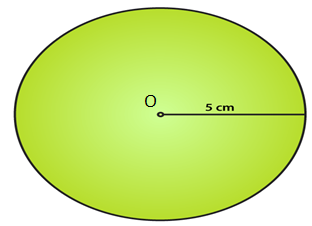We know that a semi-circle is the end point of a diameter which divides the circle into two equal parts.

No, the diameter does not determine the semi-circle and it is the end points of the diameter which finds the semi-circle or a part of the semi-circle.

7. The diameter of a circle is 14 cm, find its radius.

Solution:

It is given that

Diameter of a circle = 14 cm

We know that

Radius of a circle = Diameter / 2

By substituting the values

Radius of a circle = 14/2 = 7 cm.

8. Given a circle with centre O and radius 2.5 cm, what is the length of the longest chord of the circle.

Solution:

We know that the diameter of a circle is its longest chord which is twice its radius.

So the length of the longest chord of the circle = 2 (2.5) = 5 cm.

9. Fill in the blanks:

(i) The diameter of a circle is ……. times its radius.

(ii) The diameter of a circle is the ……. chord of the circle.

(iii) The diameter of a circle pass through ……

(iv) A chord of a circle is a line segment with its end points on the ……

(v) If we join any two points on a circle by a line segment, we obtain …… of the circle.

(vi) A radius of a circle is a line segment with one end at ……. and the other end at …..

(vii) All radii of a circle are ……

(viii) The diameters of a circle are ……

(ix) The total number of diameters of a circle is …..

(x) Every point on a circle is ……. from its centre.

(xi) A chord of a circle contains exactly …… points of the circle.

(xii) A diameter is the longest …….

(xiii) Concentric circles are circles having ……

Solution:

(i) The diameter of a circle is two times its radius.

(ii) The diameter of a circle is the longest chord of the circle.

(iii) The diameter of a circle pass through its centre.

(iv) A chord of a circle is a line segment with its end points on the circle.

(v) If we join any two points on a circle by a line segment, we obtain chord of the circle.

(vi) A radius of a circle is a line segment with one end at centre and the other end at circle.

(vii) All radii of a circle are equal.

(viii) The diameters of a circle are concurrent.

(ix) The total number of diameters of a circle is infinite.

(x) Every point on a circle is equidistant from its centre.

(xi) A chord of a circle contains exactly two points of the circle.

(xii) A diameter is the longest chord.

(xiii) Concentric circles are circles having same centre.

10. In each of the following, state if the statement is true (T) or false (F):

(i) Every circle has a centre.

(ii) The centre of a circle is a point of the circle.

(iii) Any two radii of a circle make up a diameter.

(iv) Every chord of a circle is parallel to some diameter of the circle.

(v) A circle is symmetric about each of its diameters.

(vi) The diameter is twice the radius.

(vii) A radius is a chord of the circle.

(viii) Concentric circles have the same radii.

(ix) The nearer a chord to the centre of a circle, the longer is its length.

Solution:

(i) True.

(ii) False.

(iii) False.

(iv) False.

(v) True.

(vi) True.

(vii) False.

(viii) False.

(ix) True.

### Objective Type Questions page: 14.5

Mark the correct alternative in each of the following:

1. A circle of radius r cm has diameter of length
(a) r cm
(b) 2r cm
(c) 4r cm
(d) r/2 cm

Solution:

The option (b) is the correct answer.

A circle of radius r cm has diameter of length 2r cm.

2. A chord of a circle passing through its centre is equal to its
(b) diameter
(c) circumference
(d) none of these

Solution:

The option (b) is the correct answer.

A chord of a circle passing through its centre is equal to its diameter.

3. The total number of diameters of a circle is
(a) 1
(b) 2
(c) 4
(d) uncountable number

Solution:

The option (d) is the correct answer.

The total number of diameters of a circle is uncountable number.

4. By joining any two points on a circle, we obtain its
(b) diameter
(c) chord
(d) circumference

Solution:

The option (c) is the correct answer.

By joining any two points on a circle, we obtain its chord.

5. The longest chord of a circle is equal to its
(b) diameter
(c) circumference
(d) perimeter

Solution:

The option (b) is the correct answer.

The longest chord of a circle is equal to its diameter.

6. How many circles can be drawn to pass through two given points?
(a) 1
(b) 2
(c) 0
(d) As many as possible

Solution:

The option (d) is the correct answer.

Many circles can be drawn to pass through two given points.

7. How many circles can be drawn to pass through three non-collinear points?
(a) 1
(b) 2
(c) 0
(d) As many as possible

Solution:

The option (a) is the correct answer.

Only 1 circle can be drawn to pass through three non-collinear points.